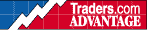HOT TOPICS LIST

INDICATORS LIST

LIST OF TOPICS

# Code For PVI & NVI Indicators

05/19/00 11:59:48 AM
by Han Kim

In a continuation of my previous article, "Positive Volume Index Or Negative Volume Index", I'm supplying the code for both indexes in Easylanguage (ELA), for TradeStation, and as an Excel spreadsheet (XLS).

Security:   N/A
Position:   N/A

 Click here to view the related article "Positive Volume Index Or Negative Volume Index".Here's how to calculate positive volume index (PVI) and negative volume index (NVI):TC = Today's closeYC = Yesterday's closeIf today's volume is greater than yesterday's volume:PVI = yesterday's PVI + (((TC - YC) / YC) * yesterday's PVI)NVI = yesterday's NVIIf today's volume is less than yesterday's volume:NVI = yesterday's NVI + (((TC - YC) / YC) * yesterday's NVI)PVI = yesterday's PVIIf today's volume equals yesterday's volume:PVI = yesterday's PVINVI = yesterday's NVI Here is the Tradestation code for PVI in Easylanguage:`Inputs: Length(255), Displace(0);Variables: PVI(100), InitValue(100);If PVI <> 0 then InitValue = 0;IF volume > volume then PVI = (PVI + InitValue) + (((close - close)/close) * (PVI + InitValue));IF volume <= volume then PVI = PVI;Plot1(PVI);If Displace >= 0 OR CurrentBar > AbsValue(Displace) Then Begin Plot2[Displace](AverageFC(PVI, Length), "PVI-MA");end;`Here is the Tradestation code for NVI in Easylanguage:`Inputs: Length(255), Displace(0);Variables: NVI(100), InitValue(100);If NVI <> 0 then InitValue = 0;IF volume < volume then NVI = (NVI + InitValue) + (((close - close)/close) * (NVI + InitValue));IF volume >= volume then NVI = NVI;Plot1(NVI);If Displace >= 0 OR CurrentBar > AbsValue(Displace) Then Begin Plot2[Displace](AverageFC(NVI, Length), "NVI-MA");end;` Click here to download the Excel spreadsheet.

Han Kim

 Comments or Questions? Article Usefulness 5 (most useful) 4 3 2 1 (least useful)

Date:�/ /Rank:�5Comment: